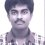Find the total number of ways of formation of numbers, which are divisible by 3, utilizing 0,1,2,3,4 and 5 without repetition.

Well the question that i was solving asked to find out the number of ways of forming five digit numbers and since I had put a lot of effort in solving it just to find out that i had misread the question, I posted the question here, so as to verify whether my approach is correct or not. Btw I am a noob at combinatorics.

So what I did was as follows:-

1 digit numbers:- 1(i.e. the number 3)

2 digit numbers:-

Lets consider that the number is formed from two digits ${ x }_{ 1 }$ & ${ x }_{ 2 }$ and as the number should be a multiple of 3 so,

${ x }_{ 1 }+{ x }_{ 2 }=3n$, where $n=1,2,3; 0{

Now, using multinomial expansion, we get $(x+{ x }^{ 2 }+{ x }^{ 3 }+{ x }^{ 4 }+{ x }^{ 5 })(1+x+{ x }^{ 2 }+{ x }^{ 3 }+{ x }^{ 4 }+{ x }^{ 5 })$ where we have to find the coefficient of ${ x }^{ 3n }, n=1,2,3$

And proceeding forward like this till the six digit numbers. Please do point out any other conditions that i might not have considered here and which would be required in further cases.5 years, 4 months ago

This discussion board is a place to discuss our Daily Challenges and the math and science related to those challenges. Explanations are more than just a solution — they should explain the steps and thinking strategies that you used to obtain the solution. Comments should further the discussion of math and science.

When posting on Brilliant:

• Use the emojis to react to an explanation, whether you're congratulating a job well done , or just really confused .
• Ask specific questions about the challenge or the steps in somebody's explanation. Well-posed questions can add a lot to the discussion, but posting "I don't understand!" doesn't help anyone.
• Try to contribute something new to the discussion, whether it is an extension, generalization or other idea related to the challenge.

MarkdownAppears as
*italics* or _italics_ italics
**bold** or __bold__ bold
- bulleted- list
• bulleted
• list
1. numbered2. list
1. numbered
2. list
Note: you must add a full line of space before and after lists for them to show up correctly
paragraph 1paragraph 2

paragraph 1

paragraph 2

[example link](https://brilliant.org)example link
> This is a quote
This is a quote
    # I indented these lines
# 4 spaces, and now they show
# up as a code block.

print "hello world"
# I indented these lines
# 4 spaces, and now they show
# up as a code block.

print "hello world"
MathAppears as
Remember to wrap math in $$ ... $$ or $ ... $ to ensure proper formatting.
2 \times 3 $2 \times 3$
2^{34} $2^{34}$
a_{i-1} $a_{i-1}$
\frac{2}{3} $\frac{2}{3}$
\sqrt{2} $\sqrt{2}$
\sum_{i=1}^3 $\sum_{i=1}^3$
\sin \theta $\sin \theta$
\boxed{123} $\boxed{123}$

Sort by:

- 5 years, 4 months ago

Just one point.. Shouldn't 0 be included since its divisible by 3 also...?

- 5 years, 4 months ago

Yeah, just forgot about that one, thanks any other thing that i might have missed.

- 5 years, 4 months ago

I'm too a noob at Combinatorics ... So I cannot help ...Regrets for that....

- 5 years, 4 months ago

- 5 years, 4 months ago